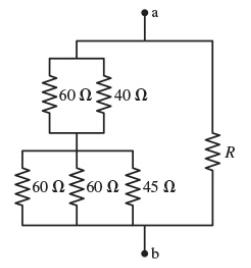# Problem: Suppose that R = 70 Ω.What is the equivalent resistance between the points a and b in Figure 1? Express your answer with the appropriate units.Rab = ____

###### FREE Expert Solution

Equivalent resistance for resistors in series:

$\overline{){{\mathbf{R}}}_{\mathbf{e}\mathbf{q}}{\mathbf{=}}{{\mathbf{R}}}_{{\mathbf{1}}}{\mathbf{+}}{{\mathbf{R}}}_{{\mathbf{2}}}{\mathbf{+}}{\mathbf{.}}{\mathbf{.}}{\mathbf{.}}{\mathbf{+}}{{\mathbf{R}}}_{{\mathbf{n}}}}$

Equivalent resistor for resistors in parallel:

$\overline{)\frac{\mathbf{1}}{{\mathbf{R}}_{\mathbf{e}\mathbf{q}}}{\mathbf{=}}\frac{\mathbf{1}}{{\mathbf{R}}_{\mathbf{1}}}{\mathbf{+}}\frac{\mathbf{1}}{{\mathbf{R}}_{\mathbf{2}}}{\mathbf{+}}{\mathbf{.}}{\mathbf{.}}{\mathbf{.}}{\mathbf{+}}\frac{\mathbf{1}}{{\mathbf{R}}_{\mathbf{n}}}}$

60Ω and 40Ω are in parallel.

1/Req1 = 1/60 + 1/40 = 1/24

Req = 24Ω

60Ω, 60Ω, and 45Ω resistors are in parallel:

1/Req2 = 1/60 + 1/60 + 1/45 = 1/18

79% (322 ratings)###### Problem Details

Suppose that R = 70 Ω.What is the equivalent resistance between the points a and b in Figure 1? Express your answer with the appropriate units.

Rab = ____

Frequently Asked Questions

What scientific concept do you need to know in order to solve this problem?

Our tutors have indicated that to solve this problem you will need to apply the Combining Resistors in Series & Parallel concept. You can view video lessons to learn Combining Resistors in Series & Parallel. Or if you need more Combining Resistors in Series & Parallel practice, you can also practice Combining Resistors in Series & Parallel practice problems.